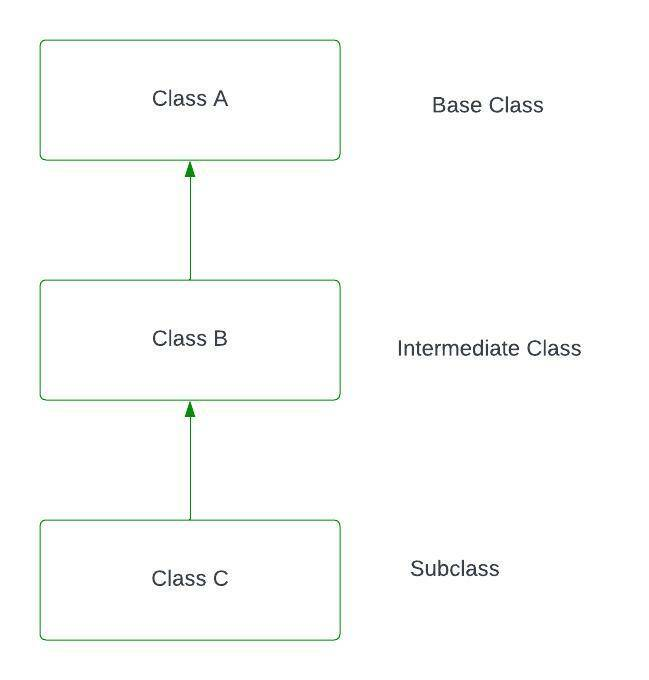GeeksforGeeks App
Open AppBrowser
Continue

# C++ Multilevel Inheritance

Multilevel Inheritance in C++ is the process of deriving a class from another derived class. When one class inherits another class it is further inherited by another class. It is known as multi-level inheritance.

For example, if we take Grandfather as a base class then Father is the derived class that has features of Grandfather and then Child is the also derived class that is derived from the sub-class Father which inherits all the features of Father.

Example:

`Base class-> Wood, Intermediate class-> furniture, subclass-> table`

## Block Diagram of Multilevel InheritanceAs shown in the diagram class B inherits property from class A and class C inherits property from class B.

Syntax:

```class A // base class
{
...........
};
class B : access_specifier A // derived class
{
...........
} ;
class C : access_specifier B // derived from derived class B
{
...........
} ;```

Know more about Access Modifiers in C++.

Example:

## C++

 `// C++ program to implement``// Multilevel Inheritance``#include ``using` `namespace` `std;` `// single base class``class` `A {``public``:``    ``int` `a;``    ``void` `get_A_data()``    ``{``        ``cout << ``"Enter value of a: "``;``        ``cin >> a;``    ``}``};` `// derived class from base class``class` `B : ``public` `A {``public``:``    ``int` `b;``    ``void` `get_B_data()``    ``{``        ``cout << ``"Enter value of b: "``;``        ``cin >> b;``    ``}``};` `// derived from class derive1``class` `C : ``public` `B {``private``:``    ``int` `c;` `public``:``    ``void` `get_C_data()``    ``{``        ``cout << ``"Enter value of c: "``;``        ``cin >> c;``    ``}` `    ``// function to print sum``    ``void` `sum()``    ``{``        ``int` `ans = a + b + c;``        ``cout << ``"sum: "` `<< ans;``    ``}``};``int` `main()``{``    ``// object of sub class``    ``C obj;` `    ``obj.get_A_data();``    ``obj.get_B_data();``    ``obj.get_C_data();``    ``obj.sum();``    ``return` `0;``}`

Output:

```Enter value of a: 4

Enter value of b: 5

Enter value of c: 9

sum: 18```

My Personal Notes arrow_drop_up